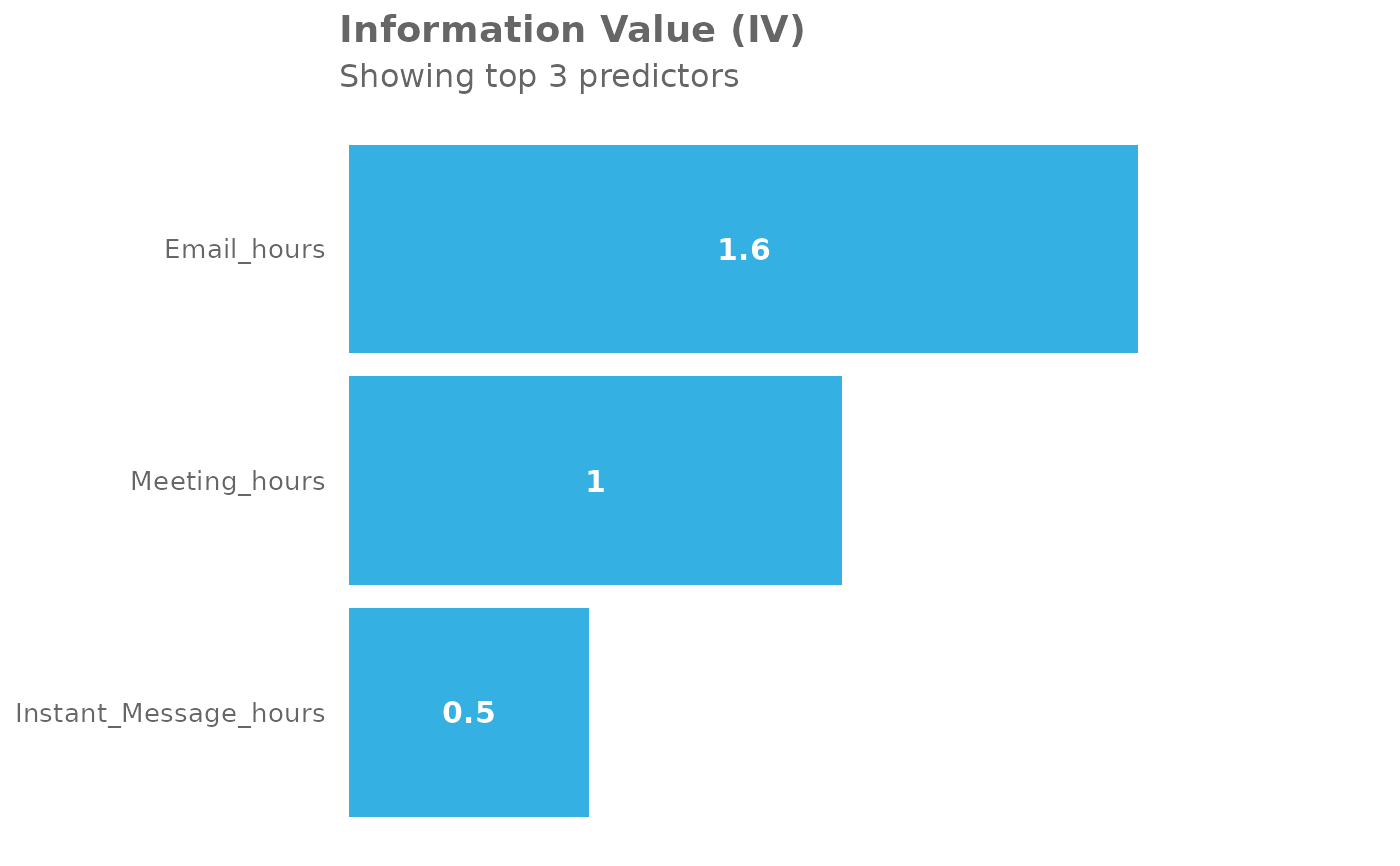Specify an outcome variable and return IV outputs. All numeric variables in the dataset are used as predictor variables.

create_IV(
data,
predictors = NULL,
outcome,
bins = 5,
siglevel = 0.05,
exc_sig = FALSE,
return = "plot"
)

## Arguments

data

A Person Query dataset in the form of a data frame.

predictors

A character vector specifying the columns to be used as predictors. Defaults to NULL, where all numeric vectors in the data will be used as predictors.

outcome

A string specifying a binary variable, i.e. can only contain the values 1 or 0.

bins

Number of bins to use, defaults to 5.

siglevel

Significance level to use in comparing populations for the outcomes, defaults to 0.05

exc_sig

Logical value determining whether to exclude values where the p-value lies below what is set at siglevel. Defaults to FALSE, where p-value calculation does not happen altogether.

return

String specifying what to return. This must be one of the following strings:

• "plot"

• "summary"

• "list"

• "plot-WOE"

• "IV"

See Value for more information.

## Value

A different output is returned depending on the value passed to the return

argument:

• "plot": 'ggplot' object. A bar plot showing the IV value of the top (maximum 12) variables.

• "summary": data frame. A summary table for the metric.

• "list": list. A list of outputs for all the input variables.

• "plot-WOE": A list of 'ggplot' objects that show the WOE for each predictor used in the model.

• "IV" returns a list object which mirrors the return in Information::create_infotables().

Other Variable Association: IV_by_period(), IV_report(), plot_WOE()

Other Information Value: IV_by_period(), IV_report(), plot_WOE()

## Examples

# Return a summary table of IV
sq_data %>%
dplyr::mutate(X = ifelse(Workweek_span > 40, 1, 0)) %>%
create_IV(outcome = "X",
predictors = c("Email_hours",
"Meeting_hours",
"Instant_Message_hours"),
return = "plot")# Return summary
sq_data %>%
dplyr::mutate(X = ifelse(Collaboration_hours > 2, 1, 0)) %>%
create_IV(outcome = "X",
predictors = c("Email_hours", "Meeting_hours"),
return = "summary")
#>        Variable       IV
#> 1 Meeting_hours 1.303955
#> 2   Email_hours 1.288803